GFG App
Open AppBrowser
Continue

# GATE | Gate IT 2007 | Question 70

Consider the regular expression R = (a + b)* (aa + bb) (a + b)*

Which deterministic finite automaton accepts the language represented by the regular expression R ?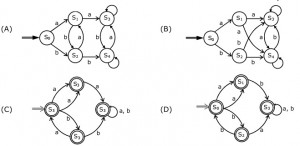(A) A
(B) B
(C) C
(D) D

Explanation: The equivalent NFA is as follows: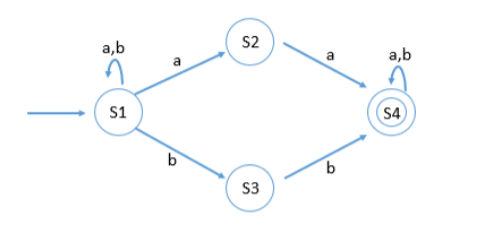Table showing transitions between all states is as follows: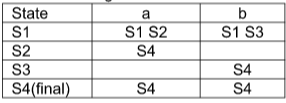The transition table can be converted to the transition table for DFA: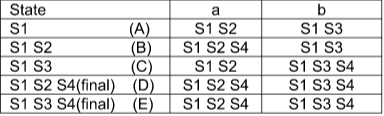Equivalent DFA for the above transition table is: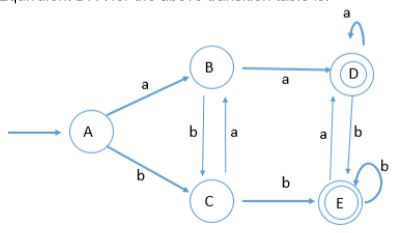Hence option (A) is the required DFA for the given regular expression.

This solution is contributed by Yashika Arora

Quiz of this Question

My Personal Notes arrow_drop_up